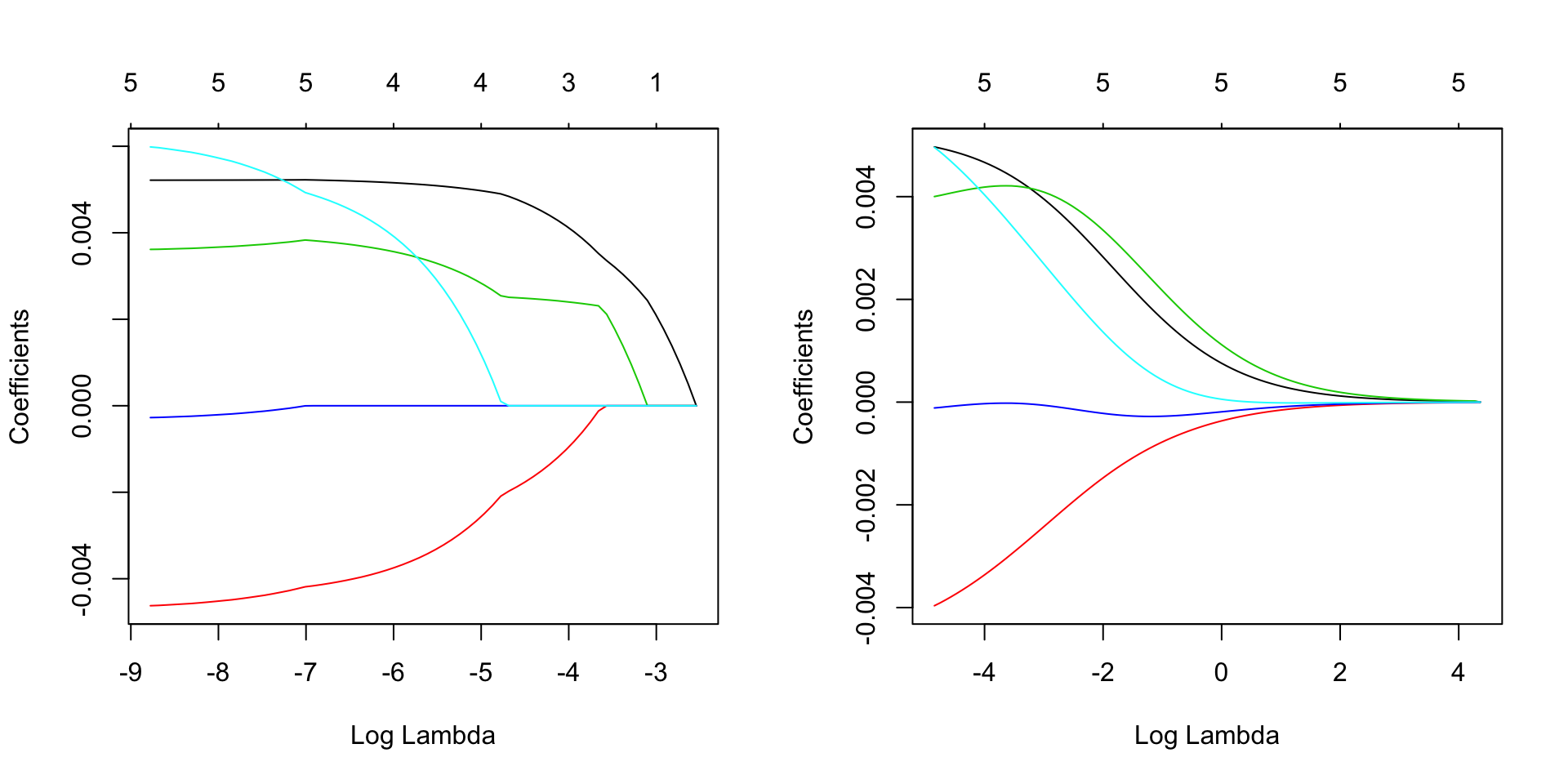We’ll reproduce the example given on page 11 of Statistical Learning with Sparsity: the Lasso and Generalizations by Hastie, Tibshirani, and Wainwright. (Code by Seth Flaxman).

``````data = read.csv("crime.txt",sep="\t")
names(data) = c("overall","violent","funding","hs","not-hs","college","college4")
library(glmnet)``````
``## Loading required package: Matrix``
``````##
## Attaching package: 'Matrix'``````
``````## The following objects are masked from 'package:base':
##
##     crossprod, tcrossprod``````
``## Loading required package: foreach``
``## Loaded glmnet 2.0-3``
``library(plotmo) ``
``## Loading required package: plotrix``
``## Loading required package: TeachingDemos``
``````data\$overall = log10(data\$overall)
par(mfrow=c(1,2))
fit.lasso = glmnet(data.matrix(data[,3:7]),data\$overall)
plot.glmnet(fit.lasso,xvar="lambda")
fit.l2 = glmnet(data.matrix(data[,3:7]),data\$overall,alpha=0)
plot.glmnet(fit.l2,xvar="lambda")````````````fit.lasso = cv.glmnet(data.matrix(data[,3:7]),data\$overall)
plot(fit.lasso)
fit.l2 = cv.glmnet(data.matrix(data[,3:7]),data\$overall,alpha=0)
plot(fit.l2)``````